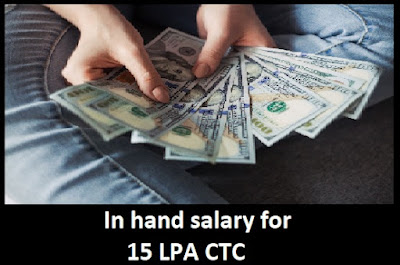# What will the in-hand salary be in India if CTC it is 15 LPA?

I will not tell the exact figure, but I will tell you the way by which you can find your in hand salary yourself. Why I am doing this, because salary breakups can be different at different levels. I am assuming 13.5 as fixed and 1.5 lakhs as Variable and we will calculate values in fixed amount.what will be in hand salary

12 % of basic salary will be deducted from the fixed component. Suppose you have 30k as basic salary, then 12% of 30000 =3600 will be deducted monthly. Now the same 12% more will be deducted as part of employer contribution. No wonder this would be a part of your CTC. If I assume 30000 as your basic salary then, annually 7200*12 = 86400 will be deducted from your CTC.

What has left now? Its 1,350,000-86,400 = 1,263,600.

Let us calculate Gratuity.

4.5% of Basic salary will go to gratuity. 1350 per month will be the gratuity and 16,200 will be annually.

What has left now? Its 1,263,600 -16200 = 1,247,400.

Now there would be some taxes based on your investment declared and the tax slabs decided by the government. I am assuming you don't have any investment like HRA, Insurance, Tax savings FD, PPF to show. In such case how tax will be imposed, let us see.

There is something call standard deduction. 50000 will be deducted from Gross Salary.

What has left now? Its 1,247,400 -50000 = 1,197,400.

You will fall under tax slab where gross salary is more than 10 lakhs.

As per new regime if your salary falls under (₹10,00,001 - ₹12,50,000).

Tax will be ₹ 1,12,500/- + 20% of total income exceeding

As per old regime if your salary exceeds ₹10,00,000.

Tax will be ₹ 1,12,500 + 30% of total income exceeding ₹10,00,000

In your case as per new regime, you need to pay 112500 + 20% of (1197400-1000000) = 151980

What has left now? Its 1,197,400 -151980= 10,45,420

After deducting taxes, we have 10,45,420 left and it will make your in hand salary. There can be professional tax which is 200 per month and 50K amount will be deducted as standard deduction. If you can declare investments and show HRA amount then less tax will be deducted. As of now the minimum salary which you can have will be 11,03,240/12= 91,937 per month.

Similarly you can calculate for old tax regime also.

So minimum salary will be this for you if basic salary is 30000. Now you can check you breakups and then replace the correct numbers to get your salary exactly.